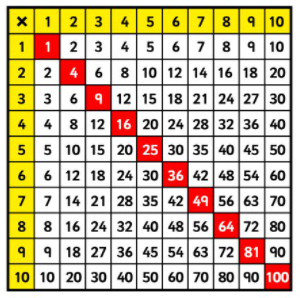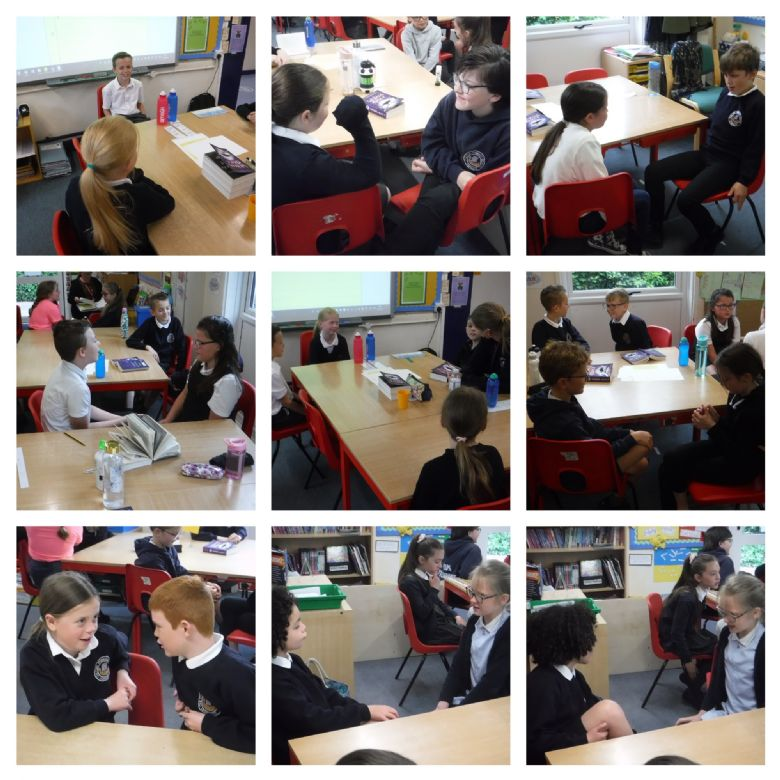# Year 4 Weekly News 14.5.21

Maths

In maths this week the children have revisited the short division method (also known as the bus stop method) for division. They have learnt how to regroup to answer questions such as 456 ÷ 3, 4 ÷ 3 is 1 remainder 1 so they have learnt to regroup the 1 just before the 5 to create 15, then 15÷3 is 5, and 6÷3 is 2, so the final answer is 152. They have also learnt that if they cannot divide by the first number, they must look at the first 2 digits and divide into that numbers e.g. 159 ÷ 3, 3 isn't divisible into 1, so we must look at the 15, which is 5 and then 9÷3 is 3 so the final answer they will get above the bus stop is 53.

The children have also learnt about square numbers, square root and prime numbers this week. They know that 82 means 8 squared and that this means 8 x 8 so 82 is 64. They have learnt that the square root is the opposite to this so the square root of 25 is 5, and the square root of 81 is 9. We have also investigated prime numbers and found out that they are numbers that are divisible by only 1 and themselves such as 2, 3, 5, 7, 11, 13. Children who do not yet know their times tables by heart have used a multiplication square like the one below to help with this learning.English

This week we have been learning to vary our sentence openers. The children have learnt how to start sentences with:

• verbs (ending in -ing is easier, ending in -ed is tricky)
• adverbs (words ending in -ly)
• similes (as....as..... /like .....)
• prepositions (words that describe position e.g. Up on the ceiling, On his head, In the centre of the room)

In guided reading this week, the children took 'the hot seat' where they answered questions in role as if they were characters from our class text 'Wizards of Once' - they really showed that they understood the story. Here they are during the hot seat activities:Love to Learn

Maths

Complete 6 of these questions:        92 =             72 =         102  =          42 =          12=

What is the square root of 4?           What is the square root of 121?            What is the square root of 9?    What is the square root of 16?

CHALLENGE:   53 =       83 =      11=

SPAG

Show me that you can write sentences with interesting sentence openers. You can write sentences about anything you like, it could be a football match, your favourite film or about Wizards of Once, it's up to you. Write one sentence that starts with a verb, one sentence that starts with an adverb, one that starts with a simile and one that starts with a preposition. Remember to use commas in the correct place.

CHALLENGE: Write 2 sentences that start with subordinating conjunctions (I SAW A WABUB).

Please can this home learning be returned by Tuesday 18th May.

Messages

Please remember to send your child in with a water bottle every day.

Please read at least 3 times a week for 10 minutes if possible.

Many thanks

Mrs Cousins and Mr Cook